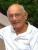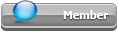## Featured Articles

Check out the latest featured articles.## File Library# What's Superficial Velocity?

This topic has been archived. This means that you cannot reply to this topic.
3 replies to this topic
|

### #1Guest_Smiley_*

Guest_Smiley_*
•• guestGuests

Posted 15 February 2005 - 06:31 PM

Hello everyone,

I looked up the sueprficial velocity definition on internet. The answer I got is that the superfical velocity is essentially the same as velocity: Volumetric Flow rate / Xross Sectional area. If superficial velocity and velocity are same, why two they differnet names?

What's the formula to calcualte the superfical vapor velocity and sueprfical liquid velocity?

Any comments are very much appreicated.

Smiley

### #2mbeychok

mbeychok

Gold Member

•• ChE Plus Subscriber
•• 364 posts

Posted 15 February 2005 - 08:30 PM

Smiley:

The velocity of a vapor or a liquid (through vessels, pipes, etc.) is equal to the volumetric flow rate divided by the cross-sectional flow area ... just as stated by the definition you found .

In some cases, as for example when expressing the flow rate of a fluid through a tower filled with packing such as rasching rings, the velocity of the fluid is calculated as if the tower were empty (i.e., the packing is ignored). In such a case, the velocity is referred to as the superficial velocity ... as differentiated from the actual velocity if the packing were not ignored.

Another exmple would be the flow of a fluid through a reactor bed of catalyst when the velocity is calculated as if the reactor were empty. Again, that velocity would be referred to as a superficial velocity.

### #3Guest_Guest_*

Guest_Guest_*
•• guestGuests

Posted 15 February 2005 - 11:17 PM

After reading your detailed and examples of superficial velocity, I have a clear understanding of it. Many thanks to you, Milton!

Smiley

### #4djack77494

djack77494

Gold Member

•• ChE Plus Subscriber
•• 1,282 posts

Posted 19 March 2005 - 02:48 AM

Smiley:
The difference between superficial velocity and what you might intuitively think of as velocity (ordinary velocity?) might be better explained by example. Lets say you have a pipe with a 1 square foot cross sectional area. If you flow 10 cubic feet per second of water through the pipe, you would have a velocity of 10 feet per second. The superficial velocity would also be 10 feet per second because the water completely fills the pipe. Similarly, if you flow 10 cubic feet per second of air through the pipe, you would have a velocity (and a superficial velocity) of 10 feet per second. So far, this is easy.

Now let's flow a mixture of 5 cubic feet per second of water AND 5 cubic feet of air through the pipe. Both phases, taken separately, would have superficial velocities of 5 feet per second (= 5 cubic feet per second/ 1 square foot). If there was no slip (both moved at the same velocity), then the actual velocities of both of the fluids would be 10 feet per second. (Without slip, each phase would occupy 50% of the pipe's cross sectional area.) With slip, the situation gets more complicated, as the actual velocity for each phase will differ.

You might want to play with some numbers to see the effects of slip on the actual velocities. Have fun.

Doug

### Similar Topics